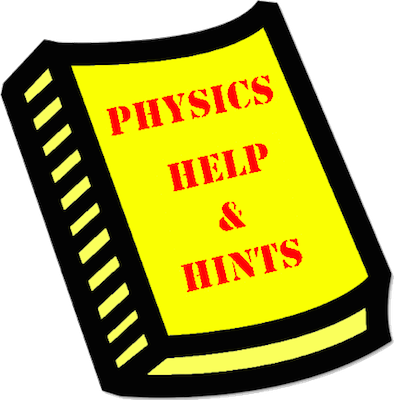# Mission NL9: Force Analysis

Mission NL9 involves the use of Newton's second law and a free-body diagram to mathematically analyze a physical situation in order to determine the net force and acceleration. The mission consists of 32 questions organized into 8 Question Groups. You must answer one question from each Question Group to complete the mission. The learning outcomes for this mission are ...

#### Learning Outcomes

• The student should be able to interpret a physics word problem to retrieve pertinent information and calculate the acceleration of an object.
• The student should be able to interpret a physics word problem to retrieve pertinent information and calculate the magnitude of an individual force.

### Launch Mission NL9

#### Getting Help

If you are not familiar with this topic, then you should first learn about the topic using our written Tutorial or our Video Tutorial:

The Physics Classroom, Newton's Laws Unit, Lesson 3, Part a

The Physics Classroom, Newton's Laws Unit, Lesson 3, Part c

The Physics Classroom, Newton's Laws Unit, Lesson 3, Part d

#### Video Tutorials:

Determining Acceleration from Force Information

Determining an Individual Force Value

####Question-Specific Help

Each Question Group has its own Help page with information specific to the question. You can access the Help page from within the mission by tapping on the Help Me! icon (textbook). For your convenience, links to those pages are provided below: